Constructing a triangle using SSS congruency criteria

Practical Geometry
Serial order wise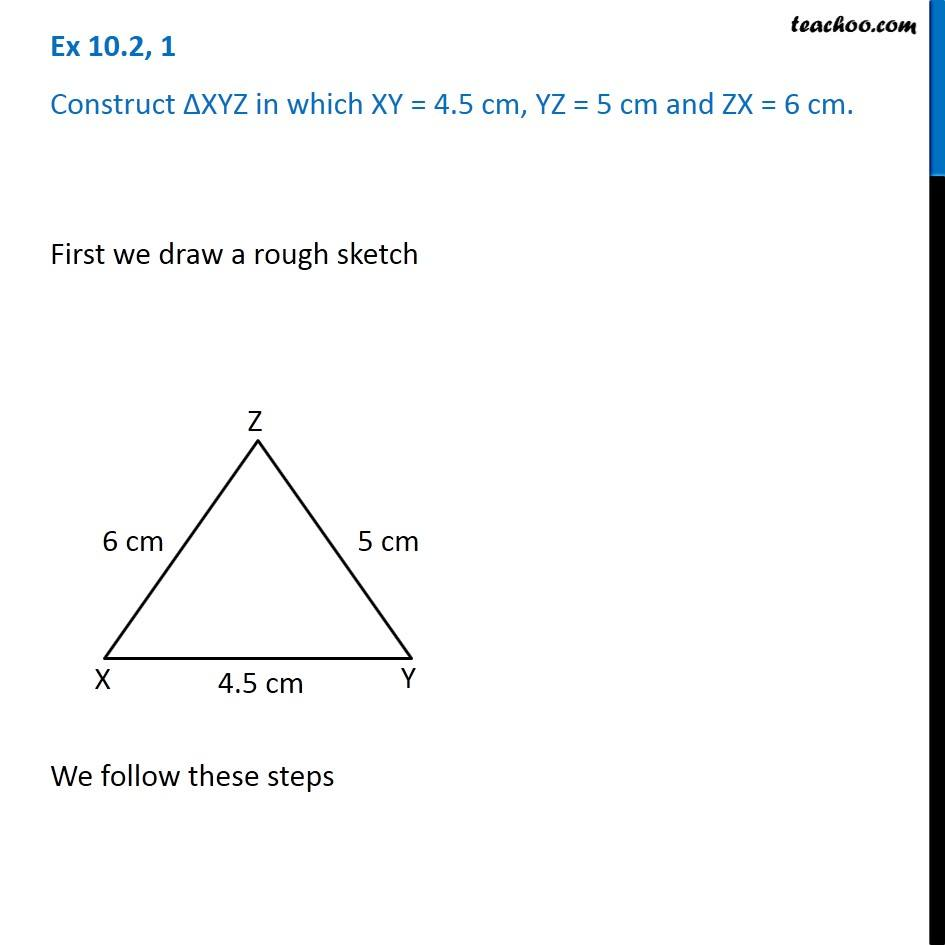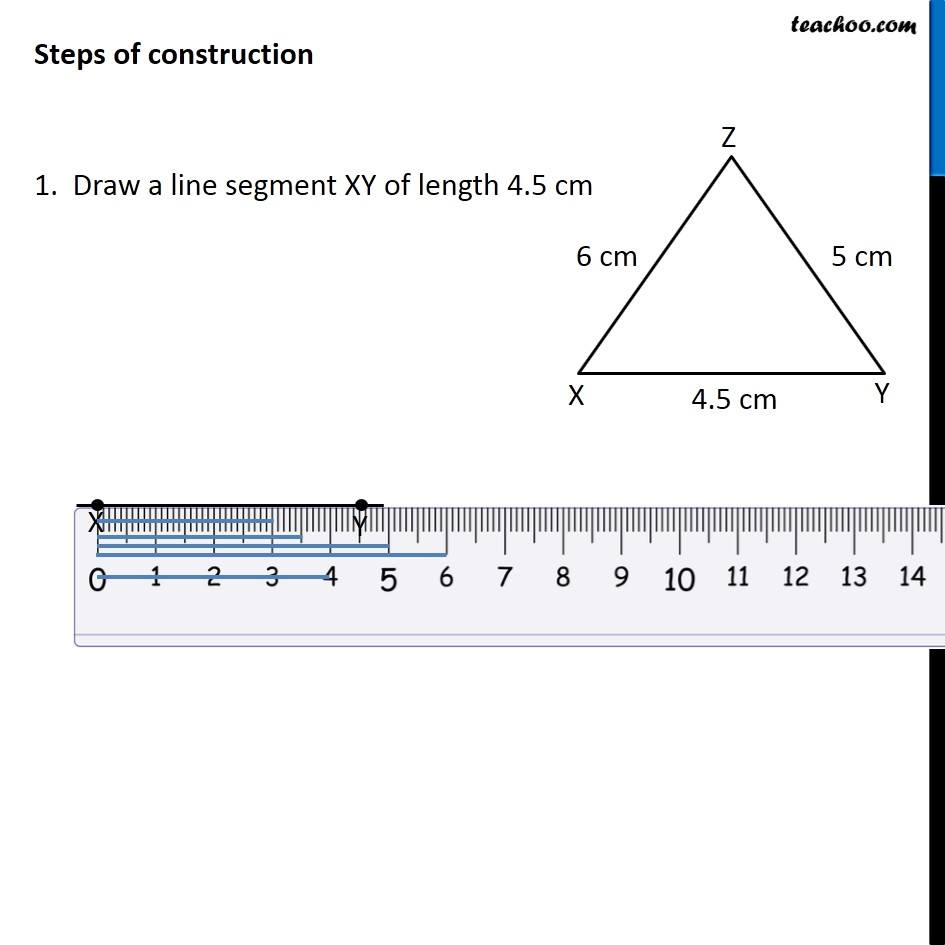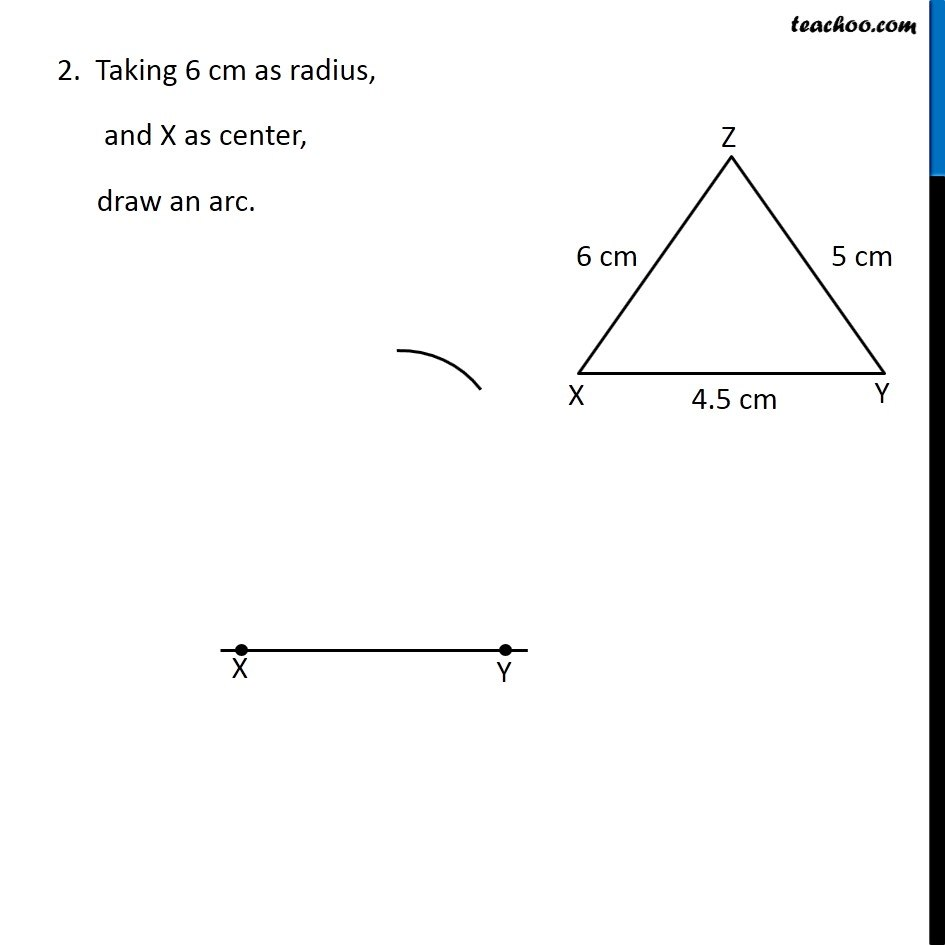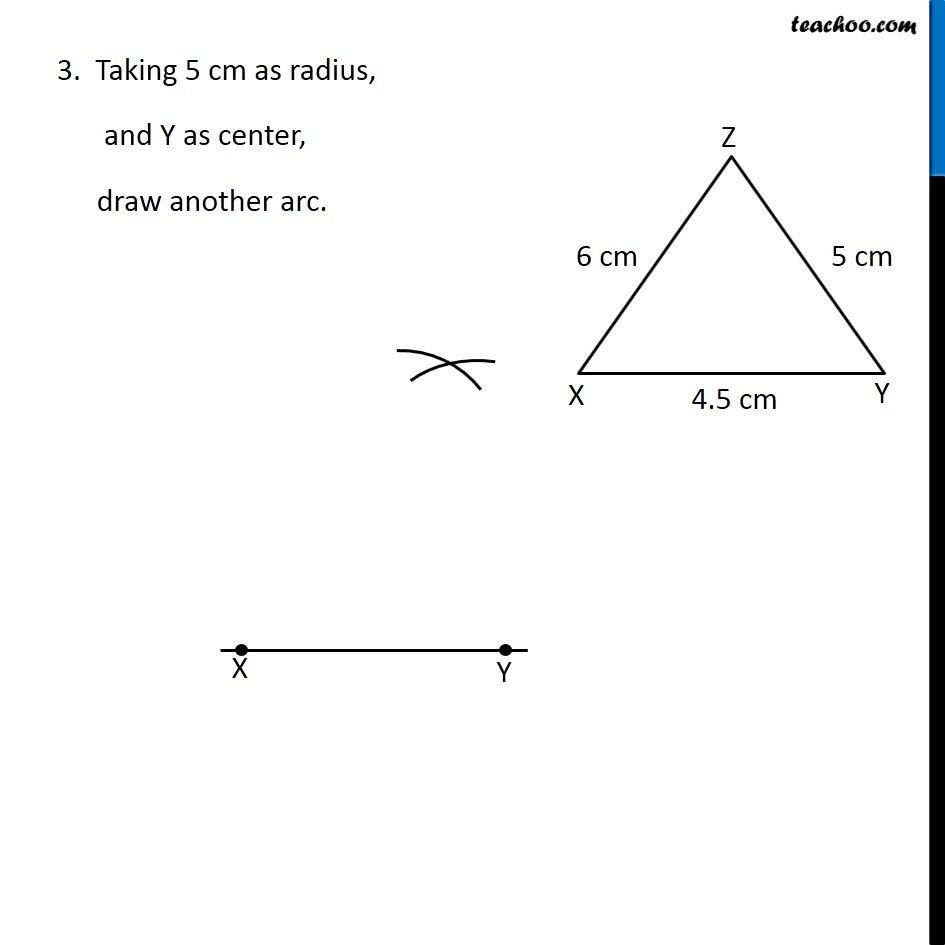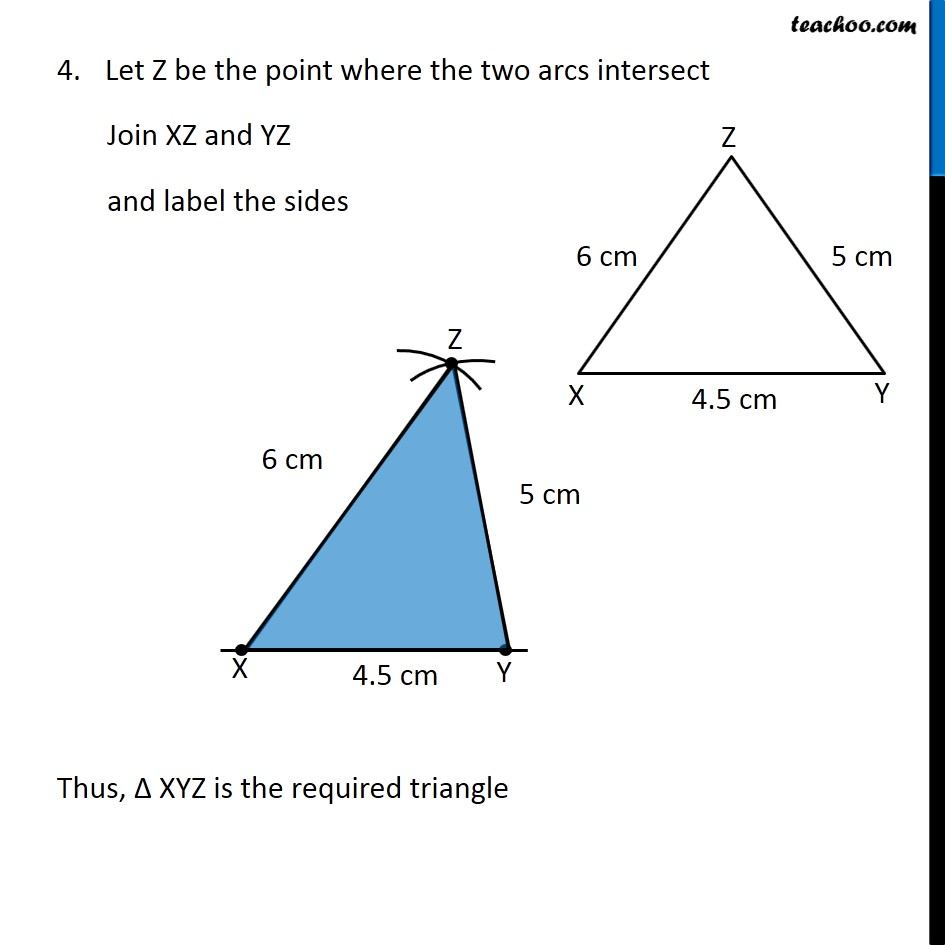Learn in your speed, with individual attention - Teachoo Maths 1-on-1 Class

### Transcript

Question 1 Construct ∆XYZ in which XY = 4.5 cm, YZ = 5 cm and ZX = 6 cm. First we draw a rough sketch We follow these steps Steps of construction 1. Draw a line segment XY of length 4.5 cm 2. Taking 6 cm as radius, and X as center, draw an arc. 3. Taking 5 cm as radius, and Y as center, draw another arc. Let Z be the point where the two arcs intersect Join XZ and YZ and label the sides Thus, Δ XYZ is the required triangle